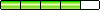# RegExLib.com - The first Regular Expression Library on the Web!

## Regular Expression Details

Title Test Find Pattern Title ^((([0-9A-Fa-f]{1,4}:){7}[0-9A-Fa-f]{1,4})|(([0-9A-Fa-f]{1,4}:){6}:[0-9A-Fa-f]{1,4})|(([0-9A-Fa-f]{1,4}:){5}:([0-9A-Fa-f]{1,4}:)?[0-9A-Fa-f]{1,4})|(([0-9A-Fa-f]{1,4}:){4}:([0-9A-Fa-f]{1,4}:){0,2}[0-9A-Fa-f]{1,4})|(([0-9A-Fa-f]{1,4}:){3}:([0-9A-Fa-f]{1,4}:){0,3}[0-9A-Fa-f]{1,4})|(([0-9A-Fa-f]{1,4}:){2}:([0-9A-Fa-f]{1,4}:){0,4}[0-9A-Fa-f]{1,4})|(([0-9A-Fa-f]{1,4}:){6}((\b((25[0-5])|(1\d{2})|(2[0-4]\d)|(\d{1,2}))\b)\.){3}(\b((25[0-5])|(1\d{2})|(2[0-4]\d)|(\d{1,2}))\b))|(([0-9A-Fa-f]{1,4}:){0,5}:((\b((25[0-5])|(1\d{2})|(2[0-4]\d)|(\d{1,2}))\b)\.){3}(\b((25[0-5])|(1\d{2})|(2[0-4]\d)|(\d{1,2}))\b))|(::([0-9A-Fa-f]{1,4}:){0,5}((\b((25[0-5])|(1\d{2})|(2[0-4]\d)|(\d{1,2}))\b)\.){3}(\b((25[0-5])|(1\d{2})|(2[0-4]\d)|(\d{1,2}))\b))|([0-9A-Fa-f]{1,4}::([0-9A-Fa-f]{1,4}:){0,5}[0-9A-Fa-f]{1,4})|(::([0-9A-Fa-f]{1,4}:){0,6}[0-9A-Fa-f]{1,4})|(([0-9A-Fa-f]{1,4}:){1,7}:))\$ Matches full and compressed IPv6 addresses as defined in RFC 2373 (http://www.faqs.org/rfcs/rfc2373.html). No useful captures. Various implementations require different terminators. (i.e. ^-\$ or \b-\b) FEDC:BA98:7654:3210:FEDC:BA98:7654:3210 | 1080::8:800:200C:417A | ::FFFF:129.144.52.38 FEDC::7654:3210::BA98:7654:3210 | FEDC:BA98:7654:3210 | :: Rating:Jeff Johnston Bad 1 2 3 4 5 Good

## Enter New Comment

 Title   Name   Comment   Spammers suck - we apologize. Please enter the text shown below to enable your comment (not case sensitive - try as many times as you need to if the first ones are too hard):Title: @Chetan Hanumantha
Name: Aeron
Date: 3/7/2012 9:25:44 AM
Comment:
Posted a bit late but your corrected regex doesn't match: :: and does match: 1111:2222:3333:4444:5555:6666:00.00.00.00 :1.2.3.4 :6666:1.2.3.4 :5555:6666:1.2.3.4 :4444:5555:6666:1.2.3.4

Title: Working regular expression. This regular expression fixes problems mentioned below.
Name: Chetan Hanumantha
Date: 3/12/2009 8:06:05 AM
Comment:
^( (([0-9A-Fa-f]{1,4}:){7}[0-9A-Fa-f]{1,4})| (([0-9A-Fa-f]{1,4}:){6}:[0-9A-Fa-f]{1,4})| (([0-9A-Fa-f]{1,4}:){5}:([0-9A-Fa-f]{1,4}:)?[0-9A-Fa-f]{1,4})| (([0-9A-Fa-f]{1,4}:){4}:([0-9A-Fa-f]{1,4}:){0,2}[0-9A-Fa-f]{1,4})| (([0-9A-Fa-f]{1,4}:){3}:([0-9A-Fa-f]{1,4}:){0,3}[0-9A-Fa-f]{1,4})| (([0-9A-Fa-f]{1,4}:){2}:([0-9A-Fa-f]{1,4}:){0,4}[0-9A-Fa-f]{1,4})| ( ([0-9A-Fa-f]{1,4}:){6} ((\b((25[0-5])|(1\d{2})|(2[0-4]\d)|(\d{1,2}))\b)\.){3} (\b((25[0-5])|(1\d{2})|(2[0-4]\d)|(\d{1,2}))\b) )| ( ([0-9A-Fa-f]{1,4}:){1,5}:((\b((25[0-5])|(1\d{2})|(2[0-4]\d)|(\d{1,2}))\b)\.){3}(\b((25[0-5])|(1\d{2})|(2[0-4]\d)|(\d{1,2}))\b))| (([0-9A-Fa-f]{1,4}:){1}:([0-9A-Fa-f]{1,4}:){0,4}((\b((25[0-5])|(1\d{2})|(2[0-4]\d)|(\d{1,2}))\b)\.){3}(\b((25[0-5])|(1\d{2})|(2[0-4]\d)|(\d{1,2}))\b))| (([0-9A-Fa-f]{1,4}:){0,2}:([0-9A-Fa-f]{1,4}:){0,3}((\b((25[0-5])|(1\d{2})|(2[0-4]\d)|(\d{1,2}))\b)\.){3}(\b((25[0-5])|(1\d{2})|(2[0-4]\d)|(\d{1,2}))\b))| (([0-9A-Fa-f]{1,4}:){0,3}:([0-9A-Fa-f]{1,4}:){0,2}((\b((25[0-5])|(1\d{2})|(2[0-4]\d)|(\d{1,2}))\b)\.){3}(\b((25[0-5])|(1\d{2})|(2[0-4]\d)|(\d{1,2}))\b))| (([0-9A-Fa-f]{1,4}:){0,4}:([0-9A-Fa-f]{1,4}:){1}((\b((25[0-5])|(1\d{2})|(2[0-4]\d)|(\d{1,2}))\b)\.){3}(\b((25[0-5])|(1\d{2})|(2[0-4]\d)|(\d{1,2}))\b))| (::([0-9A-Fa-f]{1,4}:){0,5}((\b((25[0-5])|(1\d{2})|(2[0-4]\d) |(\d{1,2}))\b)\.){3}(\b((25[0-5])|(1\d{2})|(2[0-4]\d)|(\d{1,2}))\b))| ([0-9A-Fa-f]{1,4}::([0-9A-Fa-f]{1,4}:){0,5}[0-9A-Fa-f]{1,4})| (::([0-9A-Fa-f]{1,4}:){0,6}[0-9A-Fa-f]{1,4})| (([0-9A-Fa-f]{1,4}:){1,7}:) )\$

Title: reg ex error
Name: Chetan
Date: 3/12/2009 4:53:51 AM
Comment:
This regular expression says :1.2.3.4 as valid which is wrong

Title: reg ex error
Name: Chetan
Date: 3/12/2009 4:52:29 AM
Comment:
This regular expression says :1.2.3.4 as valid which is wrong

Title: reg ex error
Name: anonymous
Date: 9/13/2006 9:56:01 AM
Comment:
error in A::A:1.1.1.1 this should be valid ipv6 address